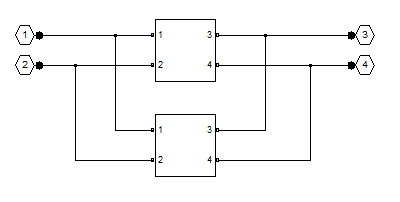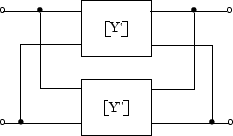# rfckt.parallel

Parallel connected network

## Description

Use the `parallel` class to represent networks of linear RF objects connected in parallel that are characterized by the components that make up the network. The following figure shows a pair of networks in a parallel configuration.## Creation

### Syntax

``h = rfckt.parallel``
``h = rfckt.parallel('Ckts',value)``

### Description

````h = rfckt.parallel` returns a parallel connected network object whose properties all have their default values.```

example

````h = rfckt.parallel('Ckts',value)` returns a cascaded network with elements specified in the name-value pair property `Ckts`.```

## Properties

expand all

Computed S-parameters, noise figure, OIP3, and group delay values, specified as rfdata.data object. `Analyzed Result` is a read-only property. For more information, see Algorithms.

Data Types: `function_handle`

Circuit objects in network, specified as a cell array of object handles. All circuits must be 2-port. By default, this property is empty.

Data Types: `char`

Object name, specified as an `1-by-N` character array. `Name` is a read-only property.

Data Types: `char`

Number of ports, specified as a positive integer. `nport`t is a read-only property. The default value is `2`.

Data Types: `double`

## Object Functions

 `analyze` Analyze RFCKT object in frequency domain `calculate` Calculate specified parameters for rfckt objects or rfdata objects `circle` Draw circles on Smith Chart `extract` Extract specified network parameters from rfckt object or data object `listformat` List valid formats for specified circuit object parameter `listparam` List valid parameters for specified circuit object `loglog` Plot specified circuit object parameters using log-log scale `plot` Plot circuit object parameters on X-Y plane `plotyy` Plot parameters of RF circuit or RF data on X-Y plane with two Y-axes `getop` Display operating conditions `polar` Plot specified object parameters on polar coordinates `semilogx` Plot RF circuit object parameters using log scale for x-axis `semilogy` Plot RF circuit object parameters using log scale for y-axis `smith` Plot circuit object parameters on Smith chart `write` Write RF data from circuit or data object to file `getz0` Calculate characteristic impedance of RFCKT transmission line object `read` Read RF data from file to new or existing circuit or data object `restore` Restore data to original frequencies `getop` Display operating conditions `groupdelay` Group delay of S-parameter object or RF filter object or RF Toolbox circuit object

## Examples

collapse all

Create a network of transmission lines connected in parallel using `rfckt.parallel`.

```tx1 = rfckt.txline; tx2 = rfckt.txline; rfplel = rfckt.parallel('Ckts',{tx1,tx2})```
```rfplel = rfckt.parallel with properties: Ckts: {[1x1 rfckt.txline] [1x1 rfckt.txline]} nPort: 2 AnalyzedResult: [] Name: 'Parallel Connected Network' ```

## Algorithms

The `analyze` method computes the S-parameters of the `AnalyzedResult` property using the data stored in the `Ckts` property as follows:

1. The `analyze` method first calculates the admittance matrix of the parallel connected network. It starts by converting each component network's parameters to an admittance matrix. The following figure shows a parallel connected network consisting of two 2-port networks, each represented by its admittance matrix,where

`$\begin{array}{l}\left[{Y}^{\prime }\right]=\left[\begin{array}{cc}{Y}_{11}{}^{\prime }& {Y}_{12}{}^{\prime }\\ {Y}_{21}{}^{\prime }& {Y}_{22}{}^{\prime }\end{array}\right]\\ \left[{Y}^{″}\right]=\left[\begin{array}{cc}{Y}_{11}{}^{\prime \text{​}\prime }& {Y}_{12}{}^{\prime \text{​}\prime }\\ {Y}_{21}{}^{\prime \text{​}\prime }& {Y}_{22}{}^{\prime \text{​}\prime }\end{array}\right]\end{array}$`

2. The `analyze` method then calculates the admittance matrix for the parallel network by calculating the sum of the individual admittances. The following equation illustrates the calculations for two 2-port circuits.

`$\left[Y\right]=\left[{Y}^{\prime }\right]+\left[{Y}^{″}\right]=\left[\begin{array}{cc}{Y}_{11}{}^{\prime }+{Y}_{11}{}^{\prime \text{​}\prime }& {Y}_{12}{}^{\prime }+{Y}_{12}{}^{\prime \text{​}\prime }\\ {Y}_{21}{}^{\prime }+{Y}_{21}{}^{\prime \text{​}\prime }& {Y}_{22}{}^{\prime }+{Y}_{22}{}^{\prime \text{​}\prime }\end{array}\right]$`

3. Finally, `analyze` converts the admittance matrix of the parallel network to S-parameters at the frequencies specified in the `analyze` input argument `freq`.

 Ludwig, R. and P. Bretchko, RF Circuit Design: Theory and Applications, Prentice-Hall, 2000.